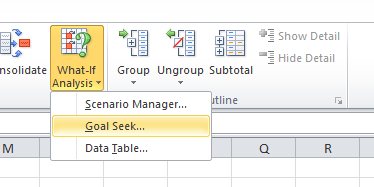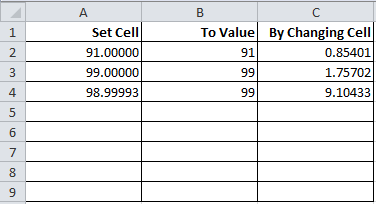# How to Use VBA to Automatically do Multiple Goal Seek (Guess and Test) Calculations?

With Goal Seek, Excel can calculate an answer by guessing and testing until it gets to the correct answer. The Goal Seek operation is quite handy when an answer we want cannot be calculated using normal formulas.The Goal Seek calculation in Excel. Useful, but very cumbersome to use if you need to do many Goal Seek operations.

However, if you want to do multiple goal seek calculations, you will normally have to do each by hand. Since each goal seek calculation has 3 inputs and will require you to wait before going onto the next calculation, it would take a lot of time to do multiple Goal Seek calculations each time something changes.

## VBA Macro Solution Code

```Sub multi_guess_and_test()

Dim ws_input As Worksheet
Dim num_rows

Set ws_input = ThisWorkbook.Worksheets("input")
num_rows = ws_input.Cells(ws_input.Rows.Count, 1).End(xlUp).Row

Dim i
Dim set_cell_range As Range, to_value_range As Range, changing_cell_range As Range
Dim to_value_val
Dim temp_val
Dim temp_range As Range

For i = 2 To num_rows 'i starts at 2 because we assume 1st row is label

Set set_cell_range = Range(ws_input.Cells(i, 1).Formula)

On Error Resume Next
Set to_value_range = Range(ws_input.Cells(i, 2).Formula)
If Err.Number <> 0 Then
to_value_val = ws_input.Cells(i, 2).Value 'find the value of cell. Even if the cell is a formula, it is ok! (Originally, goal Seek does not allow this cell to be a formula.)
End If
On Error GoTo 0

Set changing_cell_range = Range(ws_input.Cells(i, 3).Formula)

'now that all the inputs are set, perform goal seek operation
set_cell_range.GoalSeek _
Goal:=to_value_val, _
ChangingCell:=changing_cell_range

Next 'onto the next calculation!

End Sub

```

## How to Use the Macro

1. Setup the worksheet where you can in the Goal Seek parameters:
1. If you do not have a worksheet called “Input”, create a new sheet if you do not have this sheet.
2. If you already have a worksheet called “Input”, then there may be a conflict. Rename the existing sheet to something else and insert a new sheet called “Input”. Alternatively, edit the macro above if you are comfortable doing so.
2. Type in your Goal Seek parameters into the Goal Seek calculation. Notice that the column labels are exactly the same as the Goal Seek Popup Window.The Input Sheet. Each row is a separate Goal Seek calculation. Each cell is the input for the Goal Seek calculation.
1. “Set Cell” is the cell that you want to get to a certain value. Link this to the cell you are trying to set to a certain value. This cell must be a link. Can be on a different page.
2. “To Value” is the goal. Excel will try to make the cell in “Set Cell” to turn to the goal. This can be either a value or formula in the Input sheet. (For those who are familiar with the Goal Seek function, Excel by default require this input to be value, and cannot be a formula, even if the formula is linking to a value. This VBA Macro is a bit advanced in that it pulls the value of the cell, regardless of if it is a formula or not.)
3. “By Changing Cell” is the cell that Excel can change to achieve the goal. Link this to the cell that Excel can change. This cell must be a link.
4. In summary, Excel will keep on changing the cell “By Changing Cell” to get another cell “Set Cell” to your predetermined value “To Value”. Can be on a different page.
5. Your Input sheet should look something like the above. Make sure the sheet is clean and there is nothing else on it.
1. Continue. Create each new row for each new Goal Seek calculation you need. The Macro will read all rows until the end of the Input worksheet.
2. Run macro and all your Goal Seek calculations should be done automatically.